# Attention Is All You Need

### 3 Model Architecture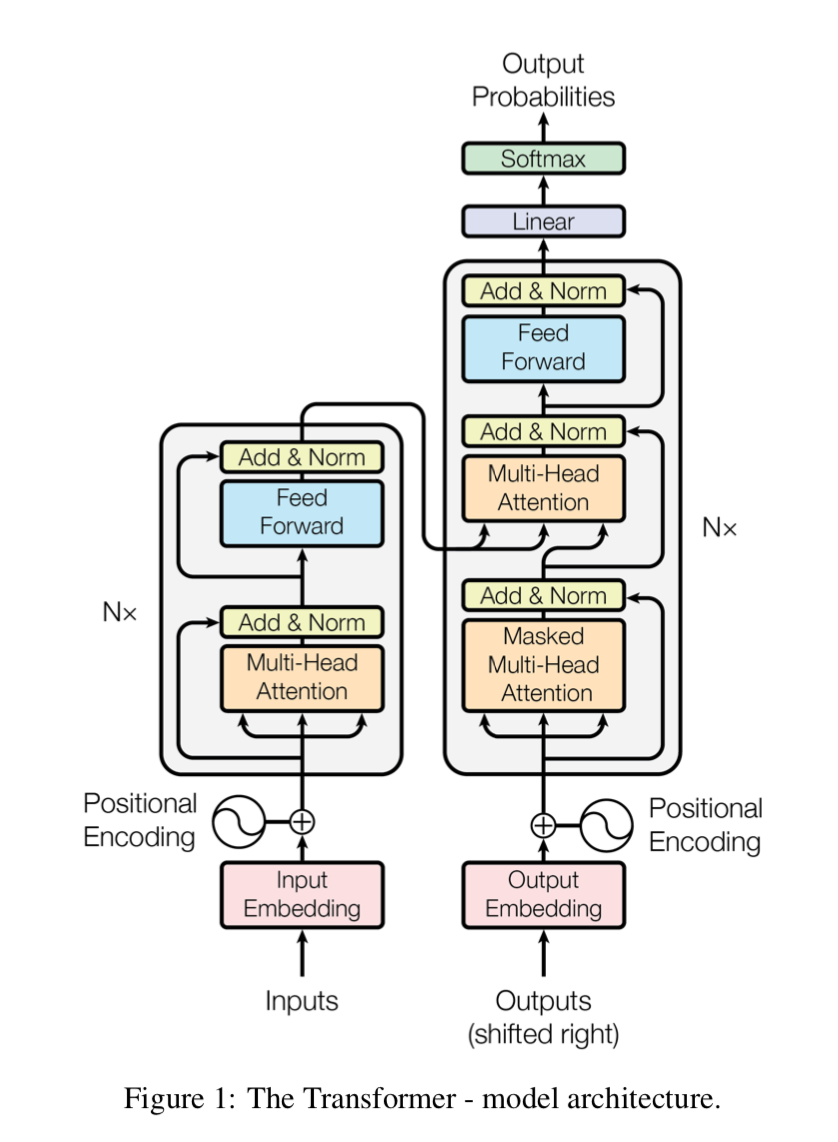Transformer整体框架如上图，分为左侧的Encoder和右侧的Decoder，都堆叠了多层。下面就模型中涉及的细节进行介绍。

#### 3.2 Attention

##### 3.2.1 Scaled Dot-Product Attention$Attention(Q,K,V)=softmax(\frac{QK^T}{\sqrt{d_k}})V$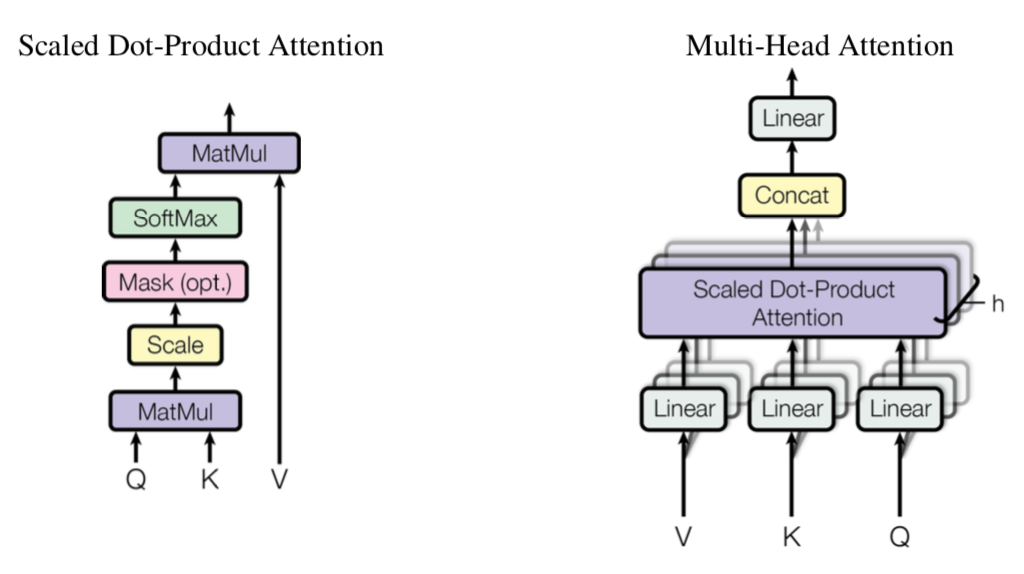$MultiHead(Q,K,V)=Concat(head_1,...,head_h)W^O \\ where \ head_i=Attention(QW_i^Q,KW_i^K,VW_i^V) \\ W_i^Q \in R^{d_{model} \times d_k},\ W_i^K \in R^{d_{model} \times d_k}, \ W_i^V \in R_{d_{model}\times d_v}, \ W^O\in R^{hd_v\times d_{model}}$

##### 3.2.3 Applications of Attention in our Model

1. 解码层中，decoder对encoder的注意力（attention）
2. encoder中的自注意力（self-attention）

#### 3.3 Position-wise Feed-Forward Networks$FFN(x)=max(0,xW_1+b_1)W_2+b_2$

#### 3.4 Embeddings and Softmax

1. Encoder和Decoder共享词向量矩阵$V$，作者特别提到在embedding层时要将这些权值乘上$\sqrt{d_{model}}$ 🤔
2. softmax层前的线性转换矩阵$U$也是与1的词向量矩阵共享权值的（相当于令$V=U$，不过有额外的bias）

#### 3.5 Positional Encoding$PE_{(pos,2i)}=sin(pos/10000^{2i/d_{model}}) \\ PE_{(pos, 2i+1)}=cos(pos/10000^{2i/d_{model}})$

### 4 Why Self-Attention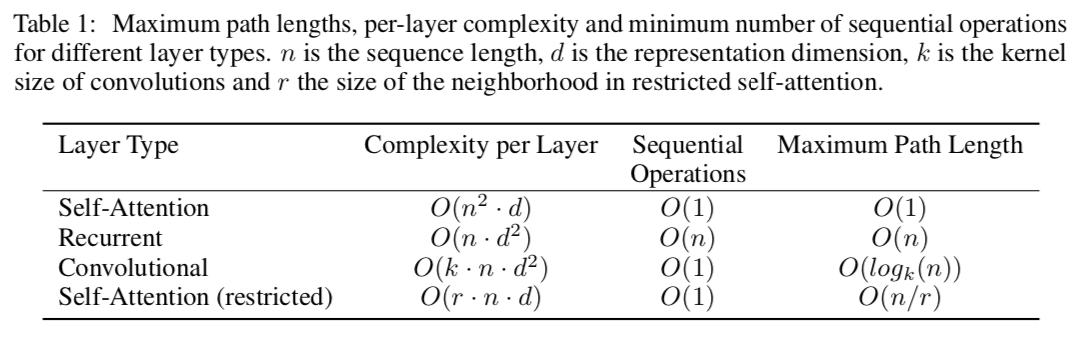1. 每层计算复杂度，这里首先介绍矩阵相乘的时间复杂度，$O(MN)=O(m\cdot n \cdot d), \\ while\ M\in R^{m\times d}, N\in R^{d\times n}$

因此，Self-Attention对应的$(1)$式的计算复杂度为$O(n^2\cdot d)$。Convolutional则是$n$个位置，每个位置有$d$$R^{k\times d}$的filter，则计算复杂度为$O(n\cdot k \cdot d^2)$。Recurrent的计算公式$H_t=\phi (...+H_{t-1}W_{dd}+b_d)$，计算复杂度$O(n\cdot d^2)$。Self-Attention (restricted)是指只对邻近$r$个token进行attention。由于$n$通常远小于$d$，所以实际上Self-Attention优势很大。

2. 可并行的运算量，用需要的最少的顺序操作的数量来衡量，即列2。

3. 长距离依赖的最长路径（越短越容易学习），Self-Attention因为任意两点间直接关联，所以路径为$O(1)$。Recurrent中序列首尾路径最长为$O(n)$。Self-Attention (restricted)首尾两个节点不能直接相连，只能通过中间一些节点间接联系在一起，路径长度$O(n/r)$。普通Convolutional同Self-Attention (restricted)，需要$O(n/k)$，如果采用空洞卷积（dilated convolutions），可以跳得更快些，为$O(log_k(n))$

另外一个好处是，self-attention具有更好的可解释性。通过可视化手段，可以让我们看到模型的关注焦点分布。

### 5 Training$lrate=d_{model}^{-0.5}\cdot min(step\_num^{-0.5}, step\_num\cdot warmup\_steps^{-1.5}), \ warmup\_steps=4000$

### 6 Results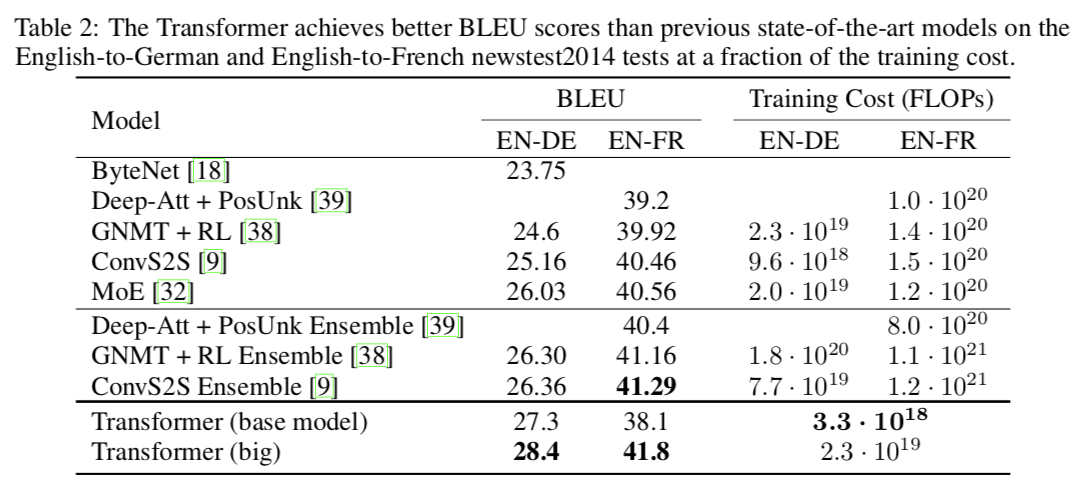可以看到在两个任务上都超越了之前的结果，并且训练代价也大为降低。论文细致分析了不同超参数设置对于模型性能的影响（分析思路值得借鉴）：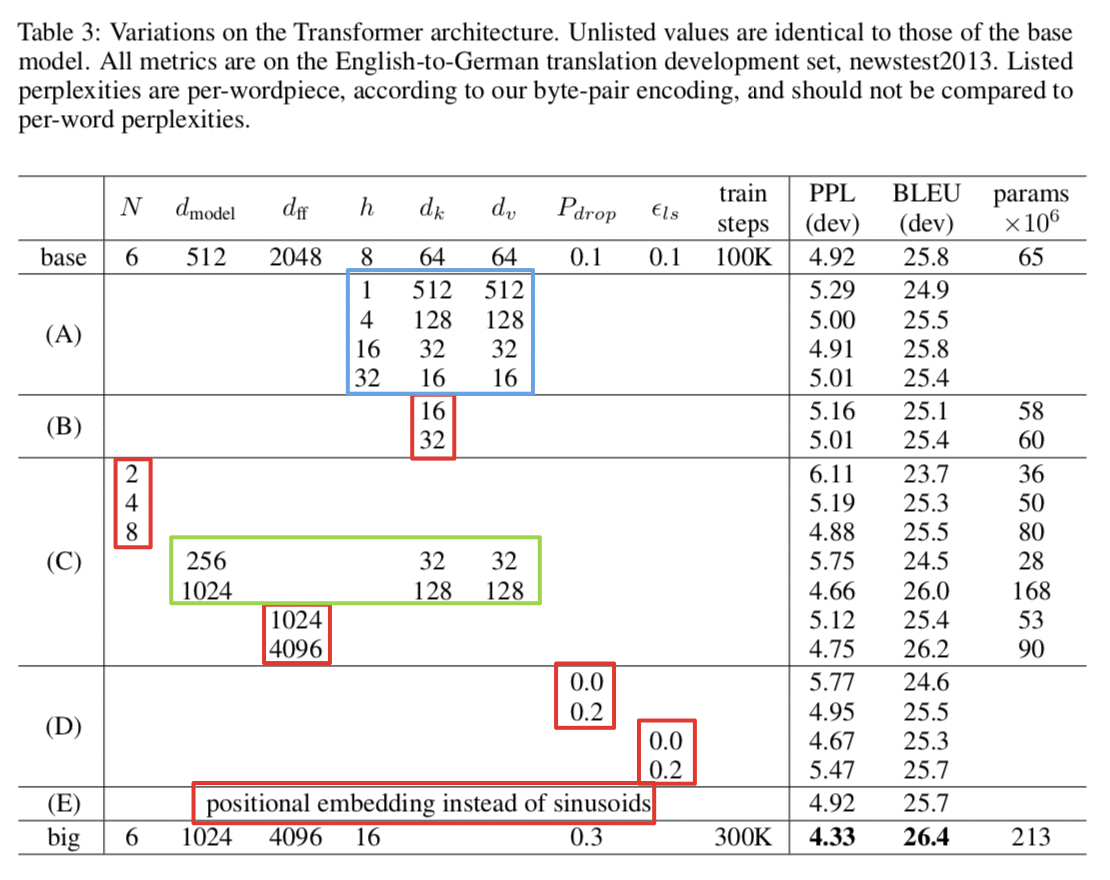红框的几组实验是控制变量的，比较容易分析，可以看到层数$N$和键大小$d_k$影响比较大（越大越好），加了dropout后提升明显，label smoothing确实提升了BLEU（但也增大了PPL），位置编码两种方式基本没有差异。蓝框和绿框是一些相关的组合参数，注意保持关系$d_{model}=h\cdot d_k$，绿框提示我们使用更高的维度，模型可以有更好的容量，蓝框告诉我们要综合权衡$h$$d_k$，最终得到big模型。

论文Appendix中给出了一些可视化例子，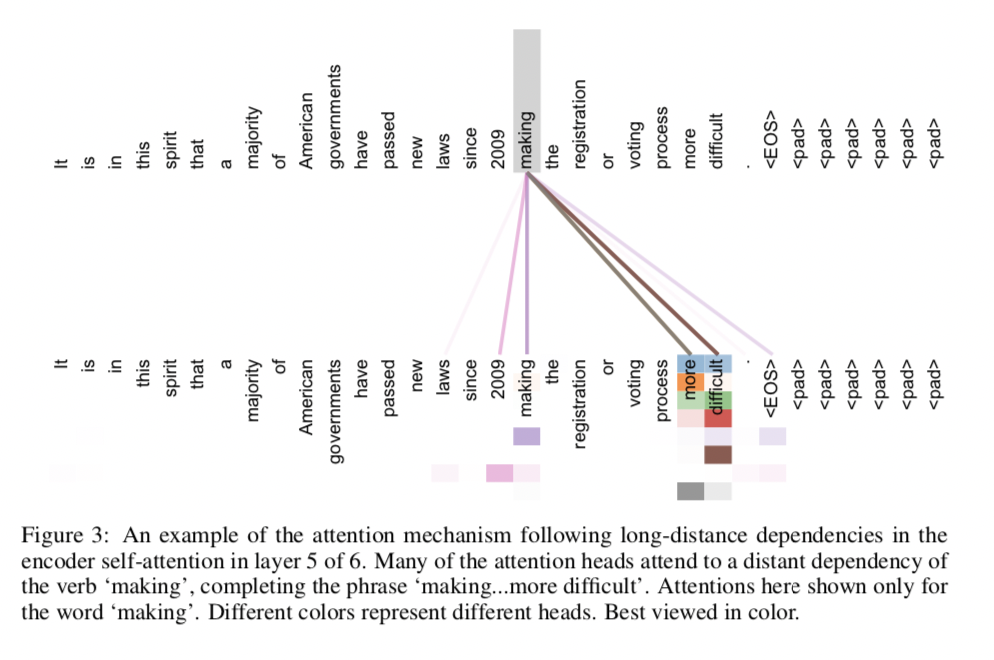making聚焦学习到了”making … more difficult”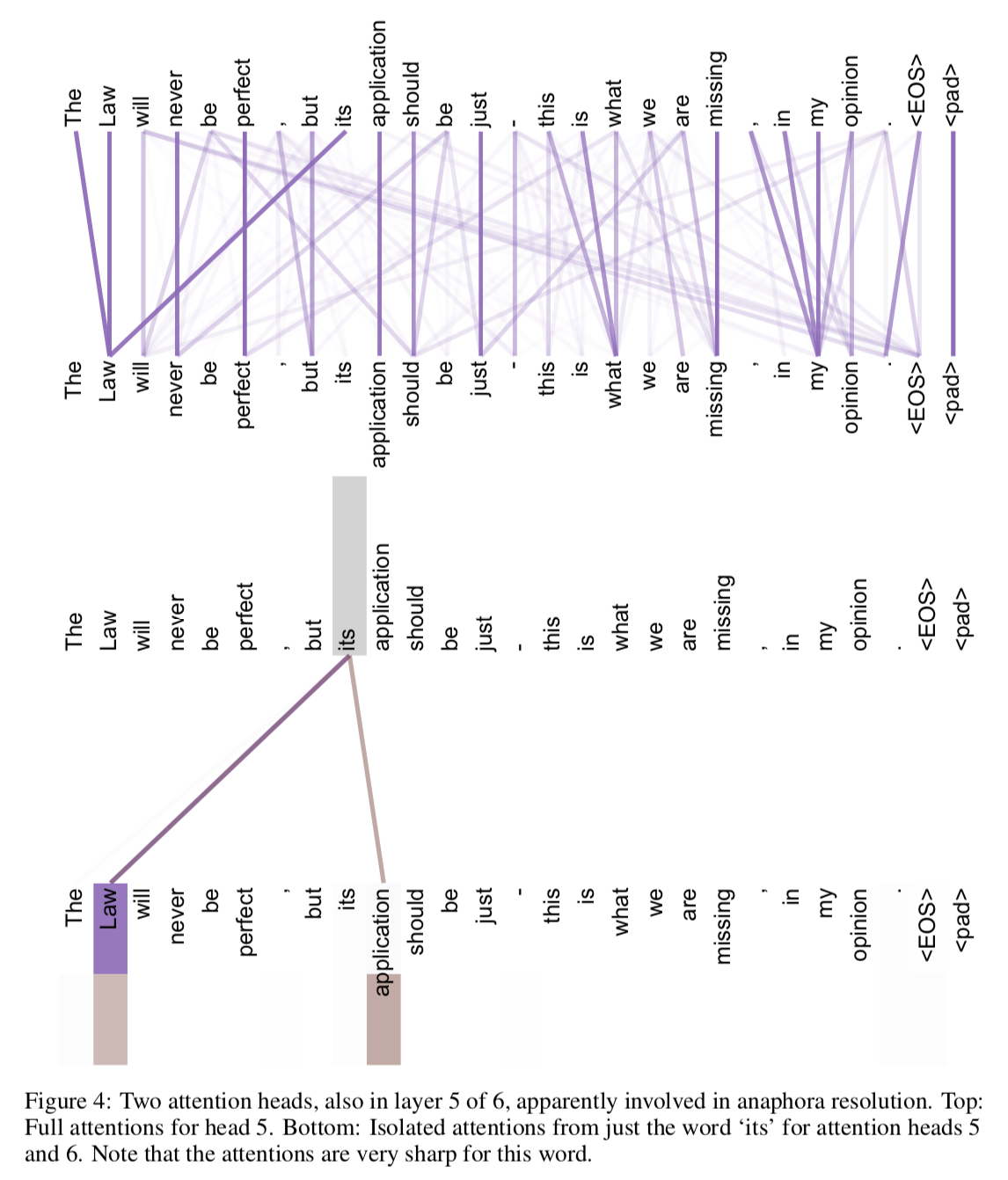单头和多头的对比，多头能够从不同的表示子空间里学习相关信息## 2 thoughts on “Attention Is All You Need”

1.admin says:

前段时间在斗鱼直播看了亚马逊AI应用科学家 Aston Zhang 的一个讲座：「动手学深度学习」番外篇——注意力机制，本来想写一篇文章总结总结的。但是，其实内容和最近的系列文章区别不大，就作罢了。不过，讲座中有几个很有意思的点，特意在这篇文章的留言评论里分享给大家。

讲座从注意力（attention出发），介绍了其基本原理、具体应用，最后还略带介绍了一下模型压缩。有一个很有趣的新颖视角——注意力机制是一种对输入分配偏好的通用池化方法，和图像领域热门已久的pooling技术联系了起来。下面是讲座中的一些笔记。

1. 注意力是什么？从《大西洋月刊》人类平均每次注意力集中时间：12秒（2000年）到8秒（2013年），不如金鱼的9秒引入——注意力是一种稀缺资源，需要合理仔细分配使用！
2. 注意力池化的几种形式（查询项：q，键项：k，值项：v）

最大池化：$max(v_i)$

平均池化：$\sum_{i=1}^{n} \frac{1}{n}v_i$

注意力机制：$\sum_{i=1}^{n}a(q,k_i)v_i$

注意力机制通常是含参数的，但也可以带来非参数模型（例如最大池化、平均池化就是两种特殊形式；注：非参数模型不是指没有参数，只是不对数据分布做假设，通常当前预测需要依赖于之前见过的数据）。Zhang举了俩个非参数模型的例子，例1，非参数回归，公式为$g(x)=E(y_i|X_i=x)$，对数据要求高，需要训练集中出现大量相同的$x$，才可使用期望进行预估，这在离散分布还有可能，连续分布基本无解。例2，Nadaraya-Waston 核回归（1964），利用$x$$X_i$的距离（核函数）作为对$y_i$进行权重平均池化，公式为$g(x)=\frac{\sum_{i=1}^{n}k(\frac{X_i-x}{h})y_i}{\sum_{i=1}^{n}{k(\frac{X_i-x}{h})}}$，这个思想和注意力机制就十分相似了，不过这里是非参数的，因为核函数是预先选定的，现在说的注意力权重一般是学习出来的。

更多内容请移步https://www.bilibili.com/video/av57405195/看视频，😂

2.admin says:

另外，注意这里用到的是层归一化方法（layer normalization），和经典的batch normalization是在mini batch数据上进行归一化不同，这个是在单个样本的不同特征维度上进行的归一化，更多解释可以看 这篇文章 ，讲的很详细。